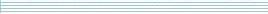| home      | people     | research     | publications    | seminars    | events     | contactCommunication Networks   | Systems Biology   | Hybrid Systems    | Machine Learning   | Dynamics & Interaction### Schools Mathematics Grand Challenge

PROBLEM 10:

Three women go into a bread shop one after another. The first woman buys half of the total number of loaves in the shop and an extra half loaf (So if there were 21 loaves in the shop, she buys 10.5 + 0.5 = 11 loaves). The second woman buys half of the loaves left in the shop and an extra half loaf. The third woman then goes in and buys half of the remaining loaves and an extra half loaf. When the third woman leaves the shop, there are no loaves left. How many loaves were in the shop to start with?

PROBLEM 11:

John, Mary, Thomas and Sheila all have mobile phones with the same company, so all four of them have different numbers. One day, while quite bored, they decide to play the following game. First, each of them subtracts the numbers of the other three people from their own number, then multiplies the three resulting differences together, and finally divides 1 by the result.

So, for instance, if the numbers of the four friends were J (for John's number), M (for Mary's number), T (for Thomas' number), S (for Sheila's number), then John would calculate

1 divided by the product (J - M)x(J - T)x(J - S),

while Mary would calculate

1 divided by the product (M - J)x(M - T)x(M - S).

If John, Mary, Thomas and Sheila add up the four answers obtained in this way, what will the sum be?

PROBLEM 12:

The number 14 is the smallest whole number with the following two properties:

1. It is bigger than 10;
2. It is smaller than the sum of the squares of its digits - (1 x 1) + (4 x 4) = 1 + 16 = 17 > 14.

What is the largest whole number satisfying these two properties?